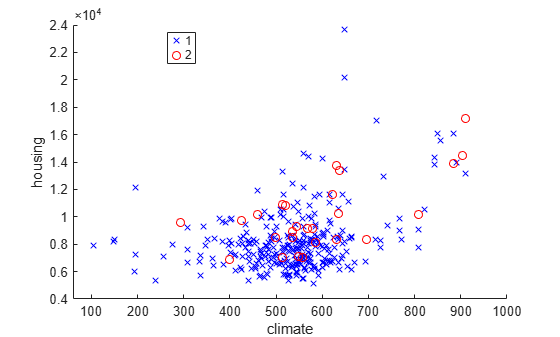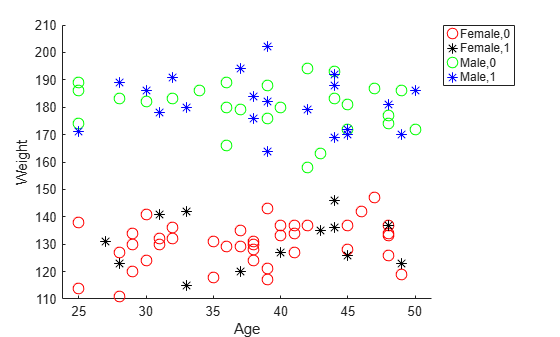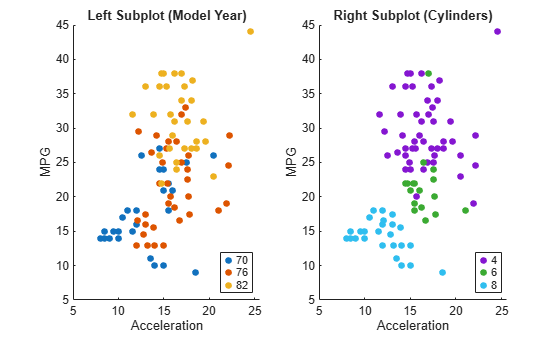# gscatter

Scatter plot by group

## Syntax

``gscatter(x,y,g)``
``gscatter(x,y,g,clr,sym,siz)``
``gscatter(x,y,g,clr,sym,siz,doleg)``
``gscatter(x,y,g,clr,sym,siz,doleg,xnam,ynam)``
``gscatter(ax,___)``
``h = gscatter(___)``

## Description

example

````gscatter(x,y,g)` creates a scatter plot of `x` and `y`, grouped by `g`. The inputs `x` and `y` are vectors of the same size.```

example

````gscatter(x,y,g,clr,sym,siz)` specifies the marker color `clr`, symbol `sym`, and size `siz` for each group.```
````gscatter(x,y,g,clr,sym,siz,doleg)` controls whether a legend is displayed on the graph. `gscatter` creates a legend by default.```

example

````gscatter(x,y,g,clr,sym,siz,doleg,xnam,ynam)` specifies the names to use for the x-axis and y-axis labels. If you do not provide `xnam` and `ynam`, and the `x` and `y` inputs are variables with names, then `gscatter` labels the axes with the variable names.```

example

````gscatter(ax,___)` uses the plot axes specified by the axes object `ax`. Specify `ax` as the first input argument followed by any of the input argument combinations in the previous syntaxes.```

example

````h = gscatter(___)` returns graphics handles corresponding to the groups in `g`.You can pass in `[]` for `clr`, `sym`, and `siz` to use their default values.```

## Examples

collapse all

Load the `carsmall` data set.

`load carsmall`

Plot the `Displacement` values on the x-axis and the `Horsepower` values on the y-axis. `gscatter` uses the variable names as the default labels for the axes. Group the data points by `Model_Year`.

`gscatter(Displacement,Horsepower,Model_Year)`Load the `discrim` data set.

`load discrim`

The data set contains ratings of cities according to nine factors such as climate, housing, education, and health. The matrix `ratings` contains the ratings information.

Plot the relationship between the ratings for climate (first column) and housing (second column) grouped by city size in the matrix `group`. Choose different colors and plotting symbols for each group.

```gscatter(ratings(:,1),ratings(:,2),group,'br','xo') xlabel('climate') ylabel('housing')```Load the `hospital` data set.

`load hospital`

Plot the ages and weights of the hospital patients. Group the patients according to their gender and smoker status. Use the `o` symbol to represent nonsmokers and the `*` symbol to represent smokers.

```x = hospital.Age; y = hospital.Weight; g = {hospital.Sex,hospital.Smoker}; gscatter(x,y,g,'rkgb','o*',8,'on','Age','Weight') legend('Location','northeastoutside')```Load the `carsmall` data set. Create a figure with two subplots and return the `axes` objects as `ax1` and `ax2`. Create a scatter plot in each set of axes by referring to the corresponding `Axes` object. In the left subplot, group the data using the `Model_Year` variable. In the right subplot, group the data using the `Cylinders` variable. Add a title to each plot by passing the corresponding `Axes` object to the `title` function.

```load carsmall color = lines(6); % Generate color values ax1 = subplot(1,2,1); % Left subplot gscatter(ax1,Acceleration,MPG,Model_Year,color(1:3,:)) title(ax1,'Left Subplot (Model Year)') ax2 = subplot(1,2,2); % Right subplot gscatter(ax2,Acceleration,MPG,Cylinders,color(4:6,:)) title(ax2,'Right Subplot (Cylinders)')```Specify marker colors using the colormap determined by the `hsv` function.

Load the Lidar scan data set which contains the coordinates of objects surrounding a vehicle, stored as a collection of 3-D points.

```load('lidar_subset.mat') loc = lidar_subset;```

To highlight the environment around the vehicle, set the region of interest to span 20 meters to the left and right of the vehicle, 20 meters in front and back of the vehicle, and the area above the surface of the road.

```xBound = 20; % in meters yBound = 20; % in meters zLowerBound = 0; % in meters```

Crop the data to contain only points within the specified region.

```indices = loc(:,1) <= xBound & loc(:,1) >= -xBound ... & loc(:,2) <= yBound & loc(:,2) >= -yBound ... & loc(:,3) > zLowerBound; loc = loc(indices,:);```

Cluster the data by using `dbscan` with pairwise distances.

```D = pdist2(loc,loc); idx = dbscan(D,2,50,'Distance','precomputed');```

Visualize the resulting clusters as a 2-D group scatter plot by using the `gscatter` function. By default, `gscatter` uses the seven MATLAB default colors. If the number of unique clusters exceeds seven, the function cycles through the default colors as needed. Find the number of clusters, and generate the corresponding number of colors by using the `hsv` function. Specify marker colors to use a unique color for each cluster.

```numGroups = length(unique(idx)); clr = hsv(numGroups); gscatter(loc(:,1),loc(:,2),idx,clr) xlabel('x') ylabel('y')```Load the `carbig` data set.

`load carbig`

Create a scatter plot comparing `Acceleration` to `MPG`. Group data points based on `Origin`.

`h = gscatter(Acceleration,MPG,Origin)`
```h = 7x1 Line array: Line (USA) Line (France) Line (Japan) Line (Germany) Line (Sweden) Line (Italy) Line (England) ```

Display the `Line` object corresponding to the group labeled `(Japan)`.

`jgroup = h(3)`
```jgroup = Line (Japan) with properties: Color: [0.9290 0.6940 0.1250] LineStyle: 'none' LineWidth: 0.5000 Marker: '.' MarkerSize: 15 MarkerFaceColor: 'none' XData: [15 14.5000 14.5000 14 19 18 15.5000 13.5000 17 ... ] YData: [24 27 27 25 31 35 24 19 28 23 27 20 22 18 20 31 32 ... ] Show all properties ```

Change the marker color for the `Japan` group to black.

`jgroup.Color = 'k';`## Input Arguments

collapse all

x-axis values, specified as a numeric vector. `x` must have the same size as `y`.

Data Types: `single` | `double`

y-axis values, specified as a numeric vector. `y` must have the same size as `x`.

Data Types: `single` | `double`

Grouping variable, specified as a categorical vector, logical vector, numeric vector, character array, string array, or cell array of character vectors. Alternatively, `g` can be a cell array containing several grouping variables (such as ```{g1 g2 g3}```), in which case observations are in the same group if they have common values of all grouping variables. Points in the same group appear on the scatter plot with the same marker color, symbol, and size.

The number of rows in `g` must be equal to the length of `x`.

Example: `species`

Example: `{Cylinders,Origin}`

Data Types: `categorical` | `logical` | `single` | `double` | `char` | `string` | `cell`

Marker colors, specified as a character vector or string scalar of short color names or a matrix of RGB triplets.

For a custom color, specify a matrix of RGB triplets. An RGB triplet is a three-element row vector whose elements specify the intensities of the red, green, and blue components of the color. The intensities must be in the range `[0,1]`; for example, ```[0.4 0.6 0.7]```.

Alternatively, you can specify some common colors by name. This table lists the named color options and the equivalent RGB triplets

Short NameRGB TripletAppearance
`'r'``[1 0 0]``'g'``[0 1 0]``'b'``[0 0 1]``'c'``[0 1 1]``'m'``[1 0 1]``'y'``[1 1 0]``'k'``[0 0 0]``'w'``[1 1 1]`Here are the RGB triplet color codes for the default colors MATLAB uses in many types of plots.

RGB TripletAppearance
`[0 0.4470 0.7410]``[0.8500 0.3250 0.0980]``[0.9290 0.6940 0.1250]``[0.4940 0.1840 0.5560]``[0.4660 0.6740 0.1880]``[0.3010 0.7450 0.9330]``[0.6350 0.0780 0.1840]`The default value for `clr` is the matrix of RGB triplets containing the MATLAB default colors.

If you do not specify enough colors for all unique groups in `g`, then `gscatter` cycles through the specified values in `clr`. If you use default values when the number of unique groups exceeds the number of default colors (7), then `gscatter` cycles through the default values as needed.

Example: `'rgb'`

Example: `[0 0 1; 0 0 0]`

Data Types: `char` | `string` | `single` | `double`

Marker symbols, specified as a character vector or string scalar of symbols recognized by the `plot` function. This table lists the available marker symbols.

ValueDescription
`'o'`Circle
`'+'`Plus sign
`'*'`Asterisk
`'.'`Point
`'x'`Cross
`'s'`Square
`'d'`Diamond
`'^'`Upward-pointing triangle
`'v'`Downward-pointing triangle
`'>'`Right-pointing triangle
`'<'`Left-pointing triangle
`'p'`Five-pointed star (pentagram)
`'h'`Six-pointed star (hexagram)
`'none'`No markers

If you do not specify enough values for all groups, then `gscatter` cycles through the specified values as needed.

Example: `'o+*v'`

Data Types: `char` | `string`

Marker sizes, specified as a positive numeric vector in points. The default value is determined by the number of observations. If you do not specify enough values for all groups, then `gscatter` cycles through the specified values as needed.

Example: `[6 12]`

Data Types: `single` | `double`

Option to include a legend, specified as either `'on'` or `'off'`. By default, the legend is displayed on the graph.

x-axis label, specified as a character vector or string scalar.

Data Types: `char` | `string`

y-axis label, specified as a character vector or string scalar.

Data Types: `char` | `string`

Axes for the plot, specified as an `Axes` or `UIAxes` object. If you do not specify `ax`, then `gscatter` creates the plot using the current axes. For more information on creating an axes object, see `axes` and `uiaxes`.

## Output Arguments

collapse all

Graphics handles, returned as an array of `Line` objects. Each `Line` object corresponds to one of the groups in `g`. You can use dot notation to query and set properties of the line objects. For a list of `Line` object properties, see Line Properties.

## Version History

Introduced before R2006a

expand all